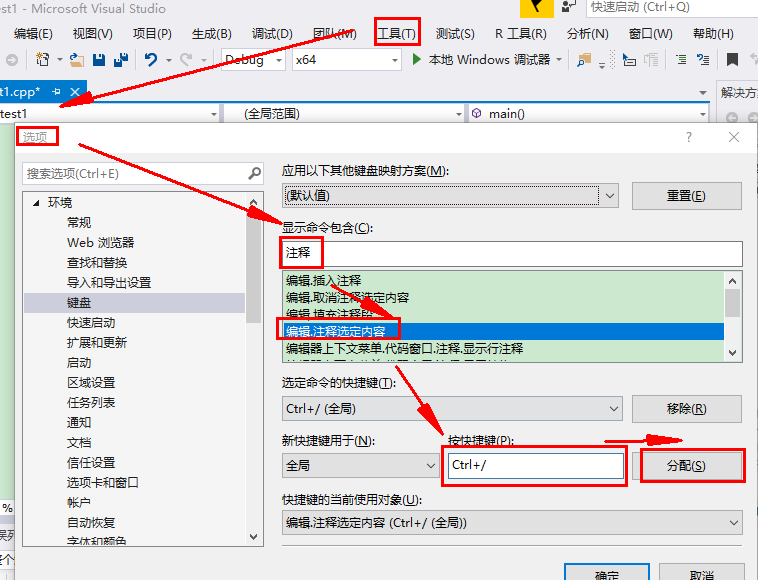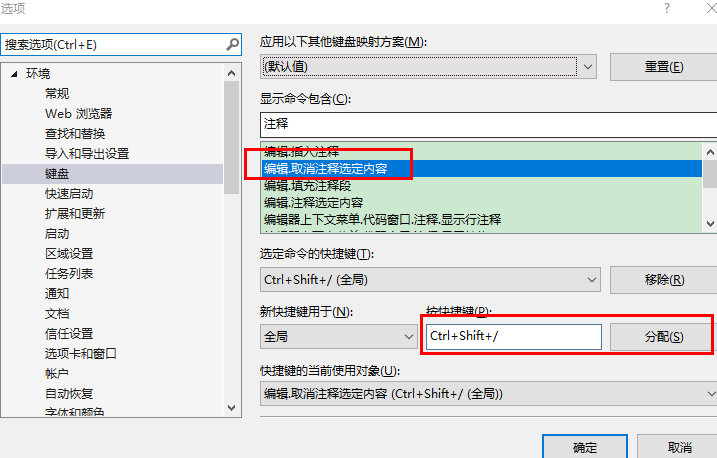• matlab根据行列标题索引表格数据 %%通过行列标题查找对应数值 path = 'C:\Users\lenovo\Desktop\matlab程序\通过表格行与列标题查找数值\table.xlsx'; table = readcell(path); % 使用readcell读取表格文件，能够...
• MATLAB矩阵结构转换及求值 这里写目录标题MATLAB矩阵结构转换及求值1.对角阵和三角阵（1）对角阵（2）三角阵2.矩阵的转置3.矩阵的逆使用矩阵求逆方法 求解线性方程组4.矩阵的行列式5.矩阵的秩和迹6.矩阵的范数7.矩阵...
MATLAB矩阵结构转换及求值
这里写目录标题MATLAB矩阵结构转换及求值1.对角阵和三角阵（1）对角阵（2）三角阵2.矩阵的转置3.矩阵的逆使用矩阵求逆方法 求解线性方程组4.矩阵的行列式5.矩阵的秩和迹6.矩阵的范数7.矩阵的条件数8.矩阵的特征值和特征向量使用求特征值的方法求解方程
表 矩阵结构变换函数及其含义

函数名
含义

diag(A)
提取矩阵A主对角线元素，产生一个具有min(m,n)个元素的列向量

diag(A,k)
提取第k条对角线的元素

diag(V)
产生一个m×m对角矩阵，其主对角线元素为向量V的元素

diag(V,k)
产生一个n×n(n=m+ |k| )对角阵，其第k条对角线的元素为向量V的元素

triu(A)
求矩阵A的上三角阵

triu(A,k)
求矩阵A的第k条对角线以上的元素

tril(A)
求矩阵A的下三角阵

tril(A,k)
求矩阵A的第k条对角线以下的元素

rot90(A,k)
将矩阵A旋转90º的k倍，按逆时针方向

fliplr(A)
对矩阵A实施左右翻转

flipud(A)
对矩阵A实施上下翻转

1.对角阵和三角阵
（1）对角阵
对角矩阵：只有对角线上有非0元素的矩阵称为对角矩阵、
数量矩阵：对角线上元素相等的对角矩阵
单位矩阵：对角线上元素全为1的对角矩阵
提取矩阵对角线元素
diag(A)：提取主对角线上的元素
diag(A,n)：提取第n条对角线上元素
注：主对角线为第0条元素，第1条对角线为主对角线往上一条，第-1条对角线为主对角线往下一条
>> A=[1 2 3;4 5 6;7 8 9]
A =

1     2     3
4     5     6
7     8     9

>> diag(A)			%提取主对角线上的元素
ans =

1
5
9

>> diag(A,-1)			%提取第-1条对角线上的元素
ans =

4
8

构造对角矩阵
设V为具有m个元素的向量。
diag(V)：产生一个m×m对角矩阵，其主对角线元素即为向量V的元素。
diag(V,k)：产生一个n×n(n=m+|k|)对角阵，其第k条对角线的元素即为向量V的元素。
>> V=[6 6 6]

V =

6     6     6

>> diag(V)			%构造以[6 6 6]为主对角线的矩阵

ans =

6     0     0
0     6     0
0     0     6

（2）三角阵
上三角阵：矩阵的对角线以下的元素全为0的一种矩阵。
下三角阵：矩阵的对角线以上的元素全为0的一种矩阵。
提取矩阵中元素
trui(A)：求矩阵A上三角阵
trui(A,K)：求矩阵A第K条对角线之上的元素
trul(A)：求矩阵A下三角阵
trul(A,K)：求矩阵A第K条对角线之下的元素
>> a=[1 2 3;4 5 6;7 8 9]

a =
1     2     3
4     5     6
7     8     9

>> trui(a,1)				%输出第一条对角线以上内容

ans =
0     2     3
0     0     6
0     0     0

2.矩阵的转置
矩阵转置运算符 :’(单引号)
>> a=[1 2 3;4 5 6;7 8 9]

a =
1     2     3
4     5     6
7     8     9

>> b=a'			%求取a的转置

b =
1     4     7
2     5     8
3     6     9

3.矩阵的逆

函数名
含义

inv(A)
求方阵A的逆矩阵

pinv(A)
求A的伪逆矩阵

矩阵的逆
a =

5     6     7
10    11    12
21    22    23

>> inv(a)

ans =

1.0e+15 *

1.8380   -2.6735    0.8355
-3.6761    5.3470   -1.6709
1.8380   -2.6735    0.8355

使用矩阵求逆方法 求解线性方程组
Ax = b
其解为：x = A’b
例如：解线性方程组
x+2y+3z=5
x+40y+9z=-2
x+8y+27z=6
clc;
A=[1,2,3;1,4,9;1,8,27]
b=[5,-2,6] '
x=inv(A)*b
x=A\b					%上行求解相当于左除

表 矩阵求值函数及其含义

函数名
含义

det(A)
求方阵A的行列式的值

rank(A)
求矩阵秩

trace(A)
求矩阵的迹

norm(A)或norm(A,2)
计算2—范数

norm(A,1)
计算1—范数

norm(A,inf)
计算∞—范数

cond(A,1)
计算1—范数下的条件数

cond(A)或cond(A,2)
计算2—范数下的条件数

cond(A,inf)
计算∞—范数下的条件数

4.矩阵的行列式
把一个方阵看作一个行列式，并对其按行列式的规则求值，这个值就称为矩阵所对应的行列式的值。
det(A)		求方阵A所对应的行列式的值。
>>a =
5     6     7
10    11    12
21    22    23

>> det(a)				%求矩阵a对应的行列式 并计算求值

ans =
-5.9846e-15

5.矩阵的秩和迹
（1）矩阵的秩
矩阵线性无关的行数与列数称为矩阵的秩。
rank(A) 求矩阵秩。
A = [2,2,-1,1;4,3,-1,2;8,5,-3,4;3,3,-2,2]
r = rank(A)
r =
4

（2）矩阵的迹
矩阵的迹等于矩阵的对角线元素之和，也等于矩阵的特征值之和。
trace(A)	求矩阵的迹。
A=[2,2,3;4,5,-6;7,8,9]
trace(A)
ans =
16

6.矩阵的范数
矩阵的范数及其计算函数
A为m×n矩阵，V为n维向量
(1) norm(A)或norm(A,2)：计算2—范数。
(2) norm(A,1)：计算1—范数。
(3) norm(A,inf)：计算∞—范数。
7.矩阵的条件数
(1) cond(A,1)：计算1—范数下的条件数。
(2) cond(A)或cond(A,2)：计算2—范数下的条件数。
(3) cond(A,inf)：计算∞—范数下的条件数。
8.矩阵的特征值和特征向量
表.求特征值与特征向量函数及其含义

函数名
含义

E=eig(A)
求全部特征值，构成向量E

[V,D]=eig(A)
求全部特征值，构成对角阵D，并求特征向量构成V的列向量

[V,D]=eig(A,‘nobalance’)
与第2种格式类似，但第2种格式中先对A作相似变换后求矩阵A的特征值和特征向量，而格式3直接求矩阵A的特征值和特征向量

使用求特征值的方法求解方程
例：3x5-7x4+5x2+2x-18=0
clc;
p=[3,-7,0,5,2,-18];
A=compan(p); 	%A的伴随矩阵
x1=eig(A)     	%求A的特征值
x2=roots(p)   	%直接求多项式p的零点



展开全文• 因为led是行列规则的，用像素和实际行列两者转换，然后取整后显示的行列重复的太多，怎么去重复的行列![图片说明](https://img-ask.csdn.net/upload/201704/11/1491881442_228215.png)
• 最近做个项目，需要将行排列的多维数组（如lua、c/c++中）与列排列的多维数组（如matlab、R、Fortune中）进行相互转换。 其实行排列、列排列是个通俗的叫法，严格上说行排列是遍历数组元素时下标从右往左递增的...
最近做个项目，需要将行排列的多维数组（如lua、c/c++中）与列排列的多维数组（如matlab、R、Fortune中）进行相互转换。

其实行排列、列排列是个通俗的叫法，严格上说行排列是遍历数组元素时下标从右往左递增的排列，而列排列是下标从左往右的排列。举例：对于int Array，如果按行排列的情况遍历，则为Array,Array,Array,Array,Array,Array,Array,Array；如果按列排列的情况遍历，则为Array,Array,Array,Array,Array,Array,Array,Array。

如何将lua的一个多维矩阵(行排列)赋值给matlab/R的多维矩阵(列排列)呢？
当然是通过c/c++。毕竟lua、matlab、R等脚本语言都是通过c支持的。另外，在进行矩阵中单个元素赋值的时候，只能通过线性下标的形式。下面给一个思路：

typedef vector<size_t> MATRIX_DIMENSION; // 数组的维度
typedef vector<size_t> MATRIX_INDEX; // 数组下标，遍历数组时使用
MATRIX_DIMENSION mat_dim = ...; // 从lua中读取过来
MATRIX_INDEX subscripts(matrixDim.size(), 0); // 下标从...开始，线性下标为0
while (subscripts没有遍历完) { // 下标当然是以从右往左的顺序递增
size_t rLinearIndice = calcSingleSubscriptOfRMatrix(mat_dim, subscripts); // 计算subscripts对应的在以列排列的数组中的线性下标
RMatrix[rLinearIndice
] = luaElement;
iterateSubscript(mat_dim, subscripts); // 让下标增一
}

bool iterateSubscript(const MATRIX_DIMENSION &dimensions, MATRIX_INDEX &subscripts)
{
MATRIX_DIMENSION::const_reverse_iterator it1;
MATRIX_INDEX::reverse_iterator it2;
for (it1 = dimensions.rbegin(), it2 = subscripts.rbegin();
it1 != dimensions.rend() && it2 != subscripts.rend();
++it1, ++it2) {
if (*it2 >= *it1)
return false;
if (*it2 < *it1-1) {
*it2 += 1;
return true;
}
else { // *it2 == *it1 - 1
//            if (it2 == subscripts.rend()-1)
//                return false;
*it2 = 0;
}
}
return true;
}

// 求以列排列的矩阵中元素的线性下标
size_t calcSingleSubscriptOfRMatrix(const MATRIX_DIMENSION &dimensions, const MATRIX_INDEX &subscripts)
{
MATRIX_INDEX vecEx;
size_t temp = 1;
vecEx.push_back(temp);
MATRIX_INDEX::const_iterator it1, it2, it3;
for (it1 = dimensions.begin(); it1 != dimensions.end()-1; ++it1) {
temp *= *it1;
vecEx.push_back(temp);
}
size_t ret = 0;
for (it2 = vecEx.begin(), it3 = subscripts.begin();
it2 != vecEx.end(), it3 != subscripts.end();
++it2, ++it3) {
ret += (*it2) * (*it3);
}
return ret;
}

At last:
希望能对需要的朋友有帮助，同时也是自己对相关知识的一个总结，方便以后用到时回忆。对于这个多维数组行列的转换，小弟还有其它一些方法，要么在时间效率上逊于本方法，要么在空间效率上逊于此，也算是走过的一个弯路，就不写出来了，有兴趣的朋友邮件或qq联系84020702@qq.com。
关于lua、R与c++混合编程的一些技术，以及量化投资技巧，也欢迎与大家讨论。第一次写博客，且小弟比较喜欢偷懒，写得比较粗略，嘿嘿，以后会越写越好的。

展开全文• ## matlab中的sub2ind函数

万次阅读 2016-05-16 09:30:41
matlab中，矩阵的存储是按列优先，sub2ind函数讲矩阵中某些元素的行列下标转换成存储的序号，即线性索引号。下面，我们举例子进行说明。
在matlab中，矩阵的存储是按列优先，sub2ind函数将矩阵中指定元素的行列下标转换成存储的序号，即线性索引号。下面，我们举例子进行说明。

1 建立一个3*4*2的矩阵
rng(0,'twister');   % Initialize random number generator.
A = rand(3, 4, 2)
A(:,:,1) =
0.8147    0.9134    0.2785    0.9649
0.9058    0.6324    0.5469    0.1576
0.1270    0.0975    0.9575    0.9706
A(:,:,2) =
0.9572    0.1419    0.7922    0.0357
0.4854    0.4218    0.9595    0.8491
0.8003    0.9157    0.6557    0.9340
将元素A（2, 1, 2）的下标转换成线性索引号

linearInd = sub2ind(size(A), 2, 1, 2)
linearInd =
14

A(2, 1, 2)            A(14)
ans =                 and =
0.4854               0.4854

2 针对这种三维的矩阵，如果缺省第三个下标，默认为1。

linearInd = sub2ind(size(A), 2, 4)
ans =
11

linearInd = sub2ind(size(A), 2, 4, 1)
ans =
11

3 假如要转换多个元素，我们可以使用一条命令完成。例子是转换5个元素下标为线性索引号。

sub2ind(size(A), 3, 3, 2);
sub2ind(size(A), 2, 4, 1);
sub2ind(size(A), 3, 1, 2);
sub2ind(size(A), 1, 3, 2);
sub2ind(size(A), 2, 4, 1);

sub2ind(size(A), [3 2 3 1 2], [3 4 1 3 4], [2 1 2 2 1])
ans =
21    11    15    19    11

%%[3 2 3 1 2], [3 4 1 3 4], [2 1 2 2 1] 分别代表行，列，纵向的下标。%%

[A(3,3,2),   A(2,4,1),   A(3,1,2),   A(1,3,2),   A(2,4,1)]
ans =
0.6557    0.1576    0.8003    0.7922    0.1576

A([21, 11, 15, 19, 11])
ans =
0.6557    0.1576    0.8003    0.7922    0.1576


展开全文• det 行列式 diag 矩阵对角元素提取、创建对角阵 diary Matlab指令窗文本内容记录 diff 数值差分、符号微分 digits 符号计算中设置符号数值的精度 dir 目录列表 disp 显示数组 display 显示对象内容的重载函数...
• （注意：matlab矩阵的行列号从1开始，python从0开始！） matlab python 创建矩阵 [1,2;4,5] mat([[1,2], [3,4]]) 将数值转换成矩阵 mat(a) 获取行列数 a.shape 取一行 a[m] 分割矩阵 a[e:f,g:h...
矩阵相关
在python里：
import numpy as np
from numpy import matrix as mat
其中a，b为矩阵；m，n为行列数
（注意：matlab矩阵的行列号从1开始，python和Armadillo从0开始！）

matlab
python
Armadillo

创建矩阵
[1,2;4,5]
mat([[1,2], [3,4]])
mat a(声明数组);a<<1<<2<<endr<<3<<4<<endr;

将数值转换成矩阵

mat(a)

获取行列数

a.shape

取一行

a[m]
a.row(m)

取第p列到q列
a(:, p:q)

a.cols(p-1, q-1)

取第p行到q行
a(p:q, : )

a.rows(p, q)

分割矩阵

a[e:f,g:h]（从e到f行，从g到h列）
a( span(s,f), span(g,h) )

矩阵元素
a(m,n)
a[m,n]
a(m,n)

零矩阵
zeros(m,n)
mat(np.zeros((m,n)))
mat a = zeros(m, n);

单位矩阵
eye(m,n)
mat(np.eye(m,n))

按行合并矩阵
cat(1,a,b)
np.vstack((a,b))
c = join_vert(a,b)

按列合并矩阵
cat(2,a,b)
np.hstack((a,b))
c = join_horiz(a,b)

行列式的值
det(a)
np.linalg.det(a)
det(a)

列和
sum(a)
a.sum(axis=0)

行和
det(a)
a.sum(axis=1)

相乘
a*b
a*b或np.dot(a,b)或np.matmul(a,b)
a%b

点乘
a.*b
multiply(a,b)
a/b

点除
a./b
a/b

求逆
inv(a)
a.I（大写的i）
a.i()或者inv(a)

转置
a’
a.T或np.transpose(a)
a.t() or trans(a)

最大值
max(a)
max(a)
a.max()

矩阵输出
a
print(a)
cout << A << endl;或者A.print(“A =”);

二范数
norm(a,2)
np.linalg.norm(a)

无穷范数
norm(a,inf)
np.linalg.norm(a, ord=np.inf)

其他计算
在python里：
import math

matlab
python

开平方
sqrt(x)
math.sqrt(x)

a的x次方
a^x
a**x

整除
/得的是精确的结果
/两边是整数时候为整除

其他
matlab有end，python没有
python有“:”，matlab没有

matlab
python

注释
%
#

不等于
~=
!=

续行
…
\

布尔运算
&，&&
and

布尔运算

or

布尔运算
~
not

函数定义
function  [output]=name(parameters)
def name(parameters):

while
while expression
while (expression):

for
for 变量=1:n
for 变量 in range(n):

if
if expression
if(expression):

在命令窗口输出
disp
print

python定义函数:
def name(para1,para2):
...
return X  #如果要返回结果的话

python
在vscode里注释的快捷键是“ctrl + /”
c++
自定义vs里的注释的快捷键：C++ 传递数组给函数
https://www.runoob.com/cplusplus/cpp-passing-arrays-to-functions.html

范数

类型
符号
计算方法

一范数
l1
绝对值（x1+x2+…+xn）

二范数
l2
sqrt(x12+x22+ … + xn2)

无穷范数
l无穷
max(绝对值（xi）)

原创，已试验过，喜欢请点赞噢:)


展开全文python numpy
• 9.2 创建和转换稀疏矩阵 123 9.3 稀疏矩阵运算 126 9.4 稀疏矩阵的特例 128 9.5 系数阵为稀疏矩阵的线性方程组 129 第10章 函数、插值和曲线拟合分析 135 10.1 MATLAB中的多项式 135 10.2 函数的零值 138 10.3 函数...
• Matlab中Notebook的应用，矩阵的建立、矩阵的拆分、特殊矩阵、矩阵算符及其表达式表示和计算，矩阵求逆，求行列式的值，矩阵特征值与特征向量计算，结构体、元胞数组，常用数学函数、画图函数应用，字符串与数值的...数值计算
• 除了Matlab教程（书样，带超连接，分章节的word版） 还有Matlab在数值计算中的实际使用和大量作图 ...4.4.1 逆矩阵、行列式及其MATLAB命令 4.4.2 方程组的逆矩阵解法及其MATLAB程序 4.5 化简与代换 4.6 其他
• ECG数据小波滤波器代码部分设计 最近在处理ECG数据的问题，关于matlab中处理ECG数据的问题也是搞了我些时间，... （行列转换） N = numel(s); %小波分解; [c,l]=wavedec(s,10,'coif4'); %小波基为coif4,分解层数为10层
• %图像旋转%X,Y为其行列数Image=imread('test.png');Image=rgb2gray(Image);angle=30;%角度任意的一个数 表示30度pai=3.14;Angle=pai*angle/180;%转换一下角度的表示方法。[X,Y]=size(Image);%原图显示subplot(2,1,1)...
• sind、cosd、tand参数均为角度制，也可使用deg2rad转换成弧度制 2—4要用点除 求行列式的值用det（） 矩阵乘积不用加点，数组乘积要加点 是35 53=3*3 左除\与右除/ A左除c即c除a或c右除a A=ones(7,7); for i=2:4 A...
• ## matlab批量处理mat

千次阅读 2017-02-16 18:24:41
这里以中国气象局的站点数据为例，转换程序如下：clc %清屏clear %清内存变量filenames = ls(‘*.txt’);%以字符串数组的形式列出该路径下所有的txt文件[m n] = size(filenames);%获取filenames的行列数for j = 1:...
• Eigen/Matlab库矩阵运算方法 Eigen库包含头文件简介： Core 有关矩阵和数组的类，有基本的线性代数（包含 三角形 和 自伴乘积 相关），还有相应对数组的操作。 Geometry 几何学的类，有关转换、平移、进位制、2D...c++ Eigen 矩阵运算
• 4.1 双下标索引： ...魔方数组：行列以及对角线的元素加起来和为一个定值 4.2 单下标索引：从第一列往下数，直到满足个数 4.3 双下标索引转换为单下标索引： 4.4 单下标转化为双下标索引： ...
• ## matlab ind2sub和sub2ind

千次阅读 2014-12-23 14:40:29
ind2sub和sub2ind是matlab中下标与索引相互转换用的两个函数。 ind代表索引（index）。sub代表下标（subscript），即行列值。 在命令窗口输入： >> A=[4 7 2 9 8;3 9 1 4 3;1 5 9 6 4;8 3 7 1 0] A = 4 7 2 ...索引
• su2ind函数是对矩阵索引号检索的函数，ans=sub2ind(size,I,J) 其中第一个参数size表示矩阵的行数和列数，I是转换矩阵的行标，J是转换矩阵的列标。I和J的行列数必须相同。最终结果ans对应I,J对应行列在整个矩阵中的...
• 4.2.1复数代数形式和极坐标形式间的相互转换程序 4.2.2求代数形式复数的倒数程序 4.2.3求任一复数平方根的程序 4.2.4求两个代数形式复数之积的程序 4.2.5求两个代数形式复数之商的程序 4.3复数运算程序2 4.3.1...
• 3.2.21 rank/det 求矩阵的秩/行列式/73 3.2.22 eig 矩阵的特征值分解/73 3.2.23 svd 矩阵的奇异值分解/74 3.2.24 trace 求矩阵的迹/75 3.2.25 norm 求向量或矩阵的范数/76 3.3 数学函数/78 3.3.1 abs 求绝对值/78 ...
• 第十一章：MATLAB符号运算11.1. 符号与数值11.1.1. 符号与数值间的转换实例-数值与符号转换11.1.2. 符号表达式与数值表达式的精度设置实例-魔方矩阵的数值解实例-稀疏矩阵的数值解实例-伴随矩阵的数值解实例-托普...
• 认真读完下面这段代码你会解决时间横坐标、双Y轴范围及图例问题 a=0; b=0; t=0:0:0 P2=load(‘D:\P2.txt’);...%将P2中第3—5列（分别为年月日）转换matlab记录时间的形式，存到第6列中 for n=1:31...
• 格式 d = size(X) %查看x的维度(行列)信息 矩阵截取 矩阵X b=X(1:m,:)取1至m行，列取全部。 c=X(:,1:n)取1至n列，行取全部。   删除矩阵中某些行 a='2 3 4 5';%定义字符串 b=str2num(a);% 将字符串转换为矩阵 tr=...
• 所有 MATLAB 变量都是多维数组，与数据类型无关。...向量的转置，用单引号(’),把行列向量之间相互转换。 想获取向量中的单个元素，用索引下标： 它跟c语言不一样，和python很像，元素个数是从1开始。 还可...
• 7 矩阵函数和数值线性代数(Matrix ...det 行列式的值 norm 矩阵或向量范数 normest 估计2 范数 null 零空间 orth 值空间 rank 秩 rref 转换为行阶梯形 trace 迹 subspace 子空间的角度 7.2 线性
• %图像旋转%X,Y为其行列数Image=imread('test.png');Image=rgb2gray(Image);angle=30;%角度任意的一个数 表示30度pai=3.14;Angle=pai*angle/180;%转换一下角度的表示方法。[X,Y]=size(Image);%原图显示subplot(2,1,1)...# matlab行列转换matlab 订阅